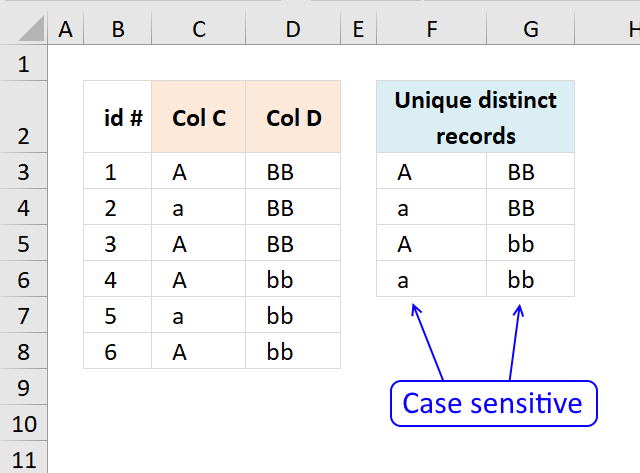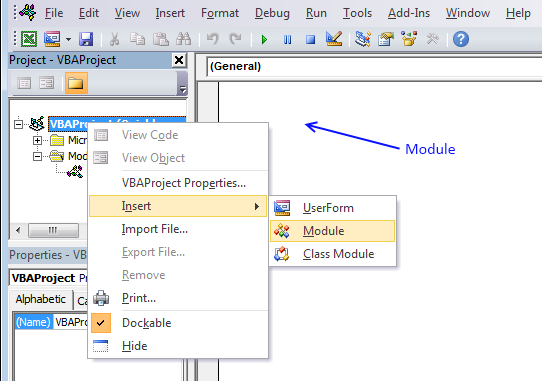Author: Oscar Cronquist Article last updated on January 18, 2019The User Defined Function demonstrated above extracts unique distinct records also considering upper and lower case letters. For example, a record containing  A and B is not the same as a record containing a and b.

A User defined Function is a custom function anyone can build, you simply add the VBA code to your workbook and you are good to go.

Array formula in cell F3:G6:

=UniqueRecords(C3:D8)

To enter an array formula, type the formula in a cell then press and hold CTRL + SHIFT simultaneously, now press Enter once. Release all keys.

The formula bar now shows the formula with a beginning and ending curly bracket telling you that you entered the formula successfully. Don't enter the curly brackets yourself.

### User defined Function Syntax

UniqueRecords(rng)

### Arguments

 Parameter Text rng Required. The range you want to use.

### Where to copy VBA code?1. Press Alt-F11 to open visual basic editor
2. Click Module on the Insert menu
3. Copy and paste above vba code
4. Exit visual basic editor

### Excel user defined function:

```'Name function and declare argument
Function UniqueRecords(rng As Variant) As Variant()
' This udf filters unique distinct records (case sensitive)
'Declare variables and data types
Dim r As Single, c As Single, temp() As Variant, k As Single
Dim rt As Single, ct As Single, a As Single, b As Single
Dim i As Single, j As Integer, iCols As Single, iRows As Single

'Save values in cell range to array variable rng (yes, same name)
rng = rng.Value
'Change size of array variable rng
ReDim temp(UBound(rng, 2) - 1, 0)
'Iterate through rows in array variable rng
For r = 1 To UBound(rng, 1)
'Iterate through rows in array variable temp
For rt = LBound(temp, 2) To UBound(temp, 2)
'Iterate through columns in array variable rng
For c = 1 To UBound(rng, 2)
' If temp value is equal rng value then increment variable a with 1
If temp(c - 1, rt) = rng(r, c) Then a = a + 1
Next c
'If a is equal to the number of columns in rng then all values in record match
If a = UBound(rng, 2) Then
a = 0
b = 0
Exit For
Else
a = 0
b = b + 1
End If
Next rt
If b = UBound(temp, 2) + 1 Then
For c = 1 To UBound(rng, 2)
temp(c - 1, UBound(temp, 2)) = rng(r, c)
Next c
ReDim Preserve temp(UBound(temp, 1), UBound(temp, 2) + 1)
b = 0
End If
Next r

k = Range(Application.Caller.Address).Rows.Count
If Range(Application.Caller.Address).Columns.Count < UBound(rng, 2) Then
MsgBox "There are more columns, extend user defined function to the right"
End If
If k < UBound(temp, 2) Then
MsgBox "There are more rows, extend user defined function down"
Else
For i = UBound(temp, 2) To k
ReDim Preserve temp(UBound(temp, 1), i)
For j = 0 To UBound(temp, 1)
temp(j, i) = ""
Next j
Next i
End If

UniqueRecords = Application.Transpose(temp)
End Function
```

### Download Excel file

* You will also get a weekly newsletter, unsubscribe anytime!